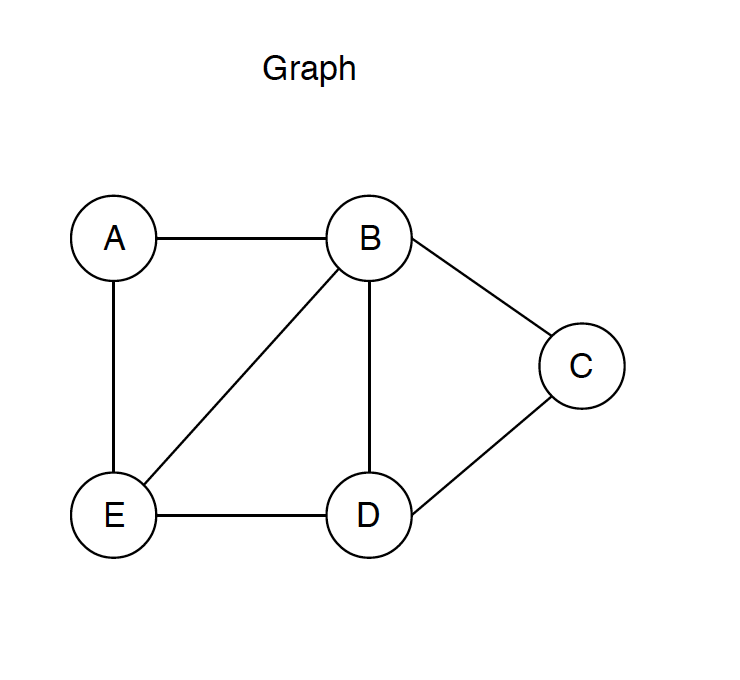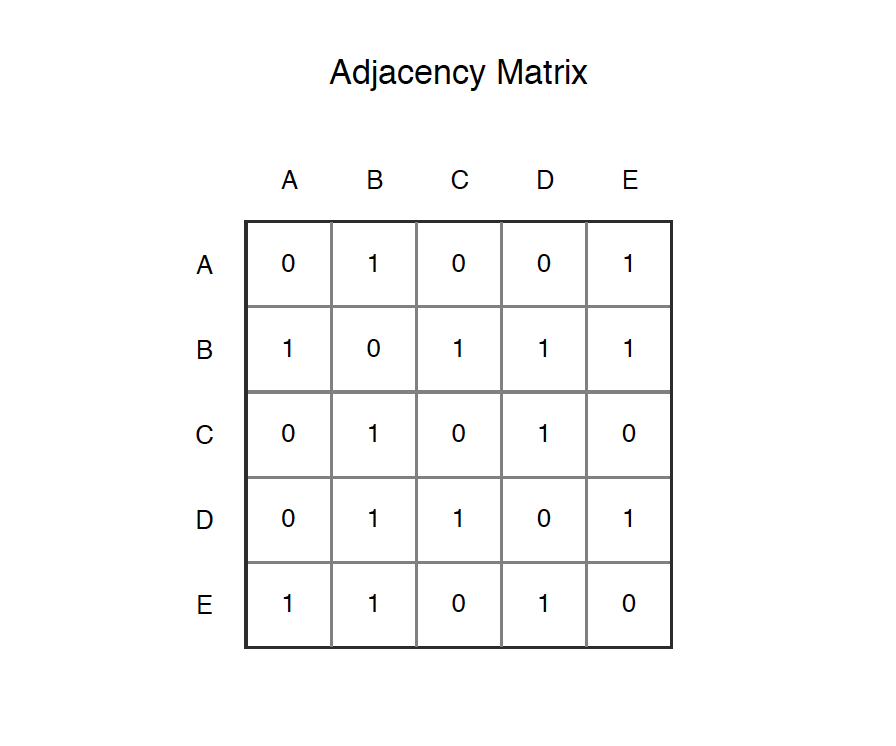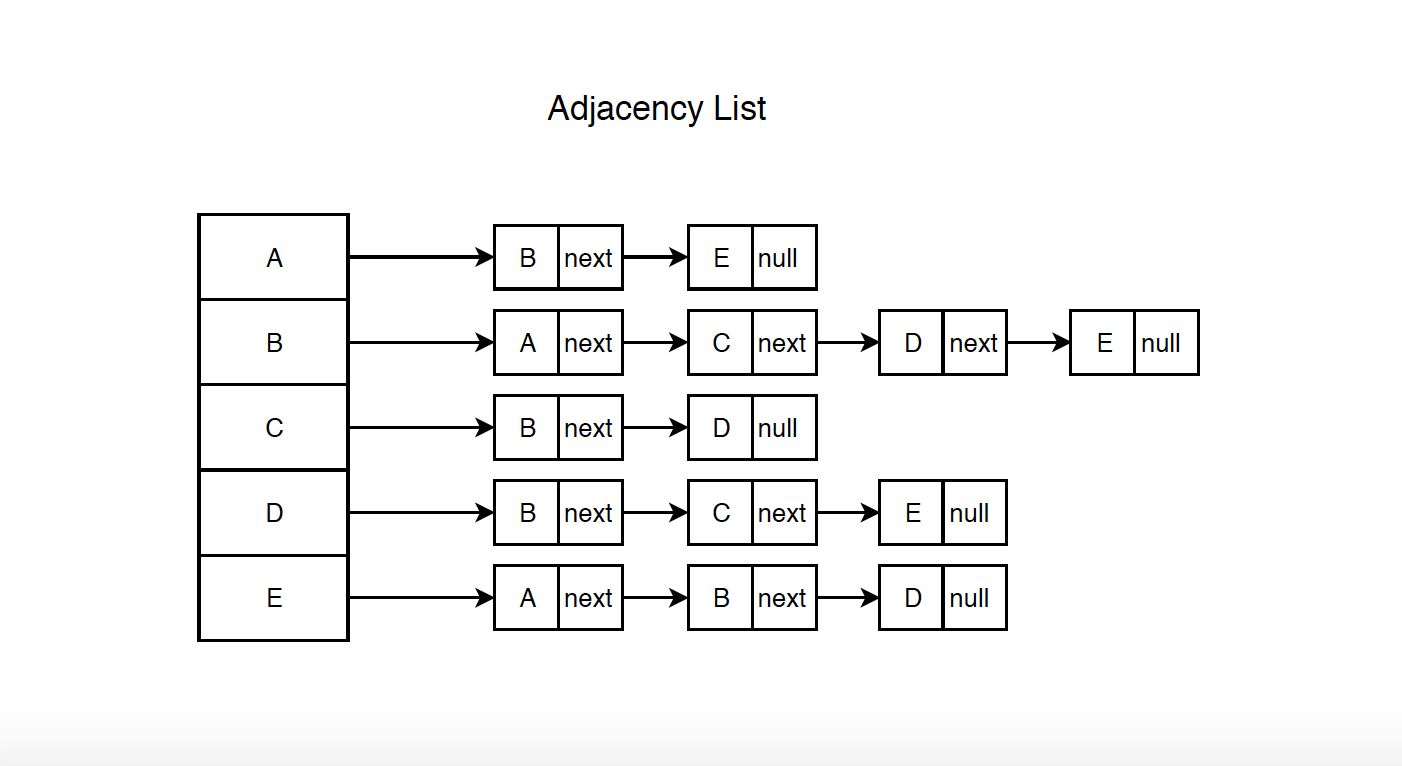# 1141. Data Structure - GraphGraph, DFS, and BFS

Implement undirected graph.

## 1. Graph

### 1.1 Definition of Graph

Graph is a data structure that consists of following two components: vertex and edge.

• A finite set of vertices, also called as nodes.
• A finite set of ordered pair of the form (u, v), also called as edge. The pair is ordered because (u, v) is not same as (v, u) in case of a directed graph(di-graph). The pair of the form (u, v) indicates that there is an edge from vertex u to vertex v. The edges may contain weight/value/cost.

Following is an example of an undirected graph with 5 vertices.### 1.2 Graph Features

• Graphs can be either directed or undirected. While directed edges are like a one-way street, undirected edges are like a two-way street.
• Graph might consist of multiple isolated subgraphs. If there is a path between every pair of vertices, it is called a ‘connected graph’.
• Graph can also have cycles (or not). An ‘acyclic graph’ is one without cycles.

## 2. Implementation of Graph

There are two most common ways to implement graph:

Adjacency Matrix is a 2D array of size V x V where V is the number of vertices in a graph. Let the 2D array be matrix[][], a slot matrix[i][j] = 1 indicates that there is an edge from vertex i to vertex j. Adjacency matrix for undirected graph is always symmetric. Adjacency Matrix is also used to represent weighted graphs. If matrix[i][j] = w, then there is an edge from vertex i to vertex j with weight w.

The adjacency matrix for the above example graph is:• Pros: Representation is easier to implement and follow. Removing an edge takes $O(1)$ time. Queries like whether there is an edge from vertex ‘u’ to vertex ‘v’ are efficient and can be done $O(1)$.
• Cons: Consumes more space $O(V^2)$. Even if the graph is sparse(contains less number of edges), it consumes the same space. Adding a vertex is $O(V^2)$ time, as you have to rebuild the matrix.

Below is the sample code which implements Graph with Adjacency Matrix.

public class Vertex {
public int index;
public String name;
public boolean visited;

public Vertex(int index, String name) {
this.index = index;
this.name = name;
this.visited = false;
}

@Override
public String toString() {
return name;
}
}

private int[][] matrix;    // adjacency matrix
private Vertex[] vertices; // array of vertices
private int size;          // current number of vertices

{
matrix = new int[capacity][capacity];
vertices = new Vertex[capacity];
size = 0;

// initialize matrix
for (int i = 0; i < capacity; i++) {
for (int j = 0; j < capacity; j++) {
matrix[i][j] = 0;
}
}
}

int index = size++;
vertices[index] = new Vertex(index, name);
}

public void addEdge(int start, int end) {
matrix[start][end] = 1;
matrix[end][start] = 1;
}
}


Adjacency List an array of lists. Size of the array is equal to the number of vertices. Let the array be vertexList[]. An entry vertexList[i] represents the list of vertices adjacent to the $i^{th}$ vertex.

Following is adjacency list representation of the above graph.• Pros: Saves space. Generally, it takes $O(V+2E)$, V is number of vertices and E is the number of edges. In the worst case, there can be $V^2$ number of edges in a graph(Every vertex connects to all other vertices) thus consuming $O(V^2)$ space. Adding a vertex is easier, just append a new node into the vertex list.
• Cons: Queries like whether there is an edge from vertex u to vertex v are not efficient and can be done $O(V)$, as you have to search one by one in the vertex list.

Below is the sample code which implements Graph with Adjacency List.

public class AdjListGraph {
private Vertex[] vertices;               // array of vertices
private int size;                        // current number of vertices

{
vertices = new Vertex[capacity];
size = 0;

// initialize array
for (int i = 0; i< vertexList.length; i++) {
}
}

int index = size++;
vertices[index] = new Vertex(index, name);
}

public void addEdge(int start, int end) {
}
}


## 3. Search In Graph

### 3.1 Search Approaches

There are two common approaches to search a graph:

• Depth-First Search: DFS is implemented with a stack.
• Breadth-First Search: BFS is implemented with a queue.

Depth-First Search(DFS)

• Rule 1: If possible, visit an adjacent unvisited vertex, mark it, and push it on the stack.
• Rule 2: If you can’t follow Rule 1, then, if possible, pop a vertex off the stack.
• Rule 3: If you can’t follow Rule 1 or Rule 2, you’re done.

• Rule 1: Visit the next unvisited vertex (if there is one) that’s adjacent to the current vertex, mark it, and insert it into the queue.
• Rule 2: If you can’t carry out Rule 1 because there are no more unvisited vertices, remove a vertex from the queue (if possible) and make it the current vertex.
• Rule 3: If you can’t carry out Rule 2 because the queue is empty, you’re done.

### 3.2 Search In Adjacency Matrix Graph

Given the above graph, the below dfs method returns [A, B, C, D, E] and the bfs method returns [A, B, E, C, D].

// dfs
private Stack<Vertex> stack = new Stack<Vertex>();
public String[] dfs() {
String[] res = new String[size];
vertices.visited = true;
int idx = 0;
res[idx++] = vertices.name;
stack.push(vertices);
while (!stack.isEmpty()) {
int index = getUnvisitedVertex(stack.peek().index);
if (index == -1) { // no unvisited neighbor
stack.pop();
} else {
vertices[index].visited = true;
res[idx++] = vertices[index].name;
stack.push(vertices[index]);
}
}

// reset vertices
for (Vertex vertex : vertices) {
vertex.visited = false;
}

return res;
}

// bfs
private Queue<Vertex> queue = new LinkedList<Vertex>();
public String[] bfs() {
String[] res = new String[size];
vertices.visited = true;
int idx = 0;
res[idx++] = vertices.name;
while (!queue.isEmpty() ) {
Vertex vertex = queue.poll();
int nextIdx = getUnvisitedVertex(vertex.index);
while (nextIdx != -1) {
vertices[nextIdx].visited = true;
res[idx++] = vertices[nextIdx].name;
nextIdx = getUnvisitedVertex(vertex.index);
}
}

// reset vertices
for (Vertex vertex : vertices) {
vertex.visited = false;
}

return res;
}

private int getUnvisitedVertex(int index) {
for (int i = 0; i < size; i++) {
if (matrix[index][i] == 1 && vertices[i].visited == false) {
return i;
}
}
return -1;
}


### 3.3 Search In Adjacency List Graph

Given the above graph, the below dfs method returns [A, B, C, D, E] and the bfs method returns [A, B, E, C, D].

// dfs
private Stack<Vertex> stack = new Stack<Vertex>();
public String[] dfs() {
String[] res = new String[size];
vertices.visited = true;
int idx = 0;
res[idx++] = vertices.name;
stack.push(vertices);
while (!stack.isEmpty()) {
int index = getUnvisitedVertex(stack.peek().index);
if (index == -1) { // no unvisited neighbor
stack.pop();
} else {
vertices[index].visited = true;
res[idx++] = vertices[index].name;
stack.push(vertices[index]);
}
}

// reset vertices
for (Vertex vertex : vertices) {
vertex.visited = false;
}
return res;
}

// bfs
private Queue<Vertex> queue = new LinkedList<Vertex>();
public String[] bfs() {
String[] res = new String[size];
vertices.visited = true;
int idx = 0;
res[idx++] = vertices.name;
while (!queue.isEmpty() ) {
Vertex vertex = queue.poll();
int nextIdx = getUnvisitedVertex(vertex.index);
while (nextIdx != -1) {
vertices[nextIdx].visited = true;
res[idx++] = vertices[nextIdx].name;
nextIdx = getUnvisitedVertex(vertex.index);
}
}

// reset vertices
for (Vertex vertex : vertices) {
vertex.visited = false;
}

return res;
}

private int getUnvisitedVertex(int index) {
for (int i = 0; i < vertexList[index].size(); i++) {
if (vertexList[index].get(i).visited == false) {
return vertexList[index].get(i).index;
}
}
return -1;
}


Bidirectional search is used to find the shortest path between a source and destination node.

## 4. Node Graph

There is one additional way to represent graph. Graph can be represented with vertex(node) only. The edges are represented with neighbor nodes, stored as a property of the node.

### 4.1 Implementation

Node.

public class Node {
public String name;
public boolean visited;
public Node[] neighbors;

public Node(String name) {
this.name = name;
this.visited = false;
}

@Override
public String toString() {
return name;
}
}


Node Graph.

public class NodeGraph {
public Node[] nodes;

public NodeGraph(int size)
{
nodes = new Node[size];
}

public void addNeighbors(int index, Node[] neighbors) {
nodes[index].neighbors = neighbors;
}
}


Both DFS and BFS approaches can be applied to Node Graph.

1) Traverse all nodes in graph with DFS.

// dfs, stack
public List<String> dfs(Node root) {
List<String> ans = new ArrayList<>();
if (root == null) {
return ans;
}
Stack<Node> stack = new Stack<Node>();
root.visited = true;
stack.push(root);
while (!stack.isEmpty()) {
Node node = stack.peek();
Node neighbor = getUnvisitedNeighbor(node);
if (neighbor == null) {
stack.pop();
} else {
neighbor.visited = true;
stack.push(neighbor);
}
}

return ans;
}
private Node getUnvisitedNeighbor(Node node) {
for (int i = 0; i < node.neighbors.length; i++) {
if (node.neighbors[i].visited == false) {
return node.neighbors[i];
}
}
return null;
}


For the DFS search, we can simplify the implementation without using stack. The dfs2 method calls itself recursively to generate the list.

// dfs, recursion
public void dfs2(Node root, List<String> list) {
if (root == null) {
return;
}
root.visited = true;
for (Node neighbor : root.neighbors) {
if (neighbor.visited == false) {
dfs2(neighbor, list);
}
}
}


2) Traverse all nodes in graph with BFS.

public List<String> bfs(Node root) {
List<String> ans = new ArrayList<>();
if (root == null) {
return ans;
}
root.visited = true;
queue.offer(root);
while (!queue.isEmpty()) {
Node node = queue.poll();
for (Node neighbor : node.neighbors) {
if (neighbor.visited == false) {
neighbor.visited = true;
queue.offer(neighbor);
}
}
}

return ans;
}